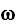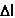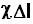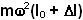Tuesday, May 6, 2008

Irodov Problem 1.142When the rod rotates at a rate, the mass experiences a centrifugal force (in the rotating reference on the rod) which extends the spring. Suppose that the spring extends by a length. When seen from the rotating reference frame on the rod, there are two forces acting on the mass, i) the tension in the springand ii) the centrifugal force. There is no acceleration for the mass along the horizontal as seen in the rotating reference frame. So we have,The work done in getting the system to this rotating state is equal to the total energy stored in the system which is a sum of i) potential energy stored in the spring and ii) the kinetic energy of the mass. Thus, the work done is,